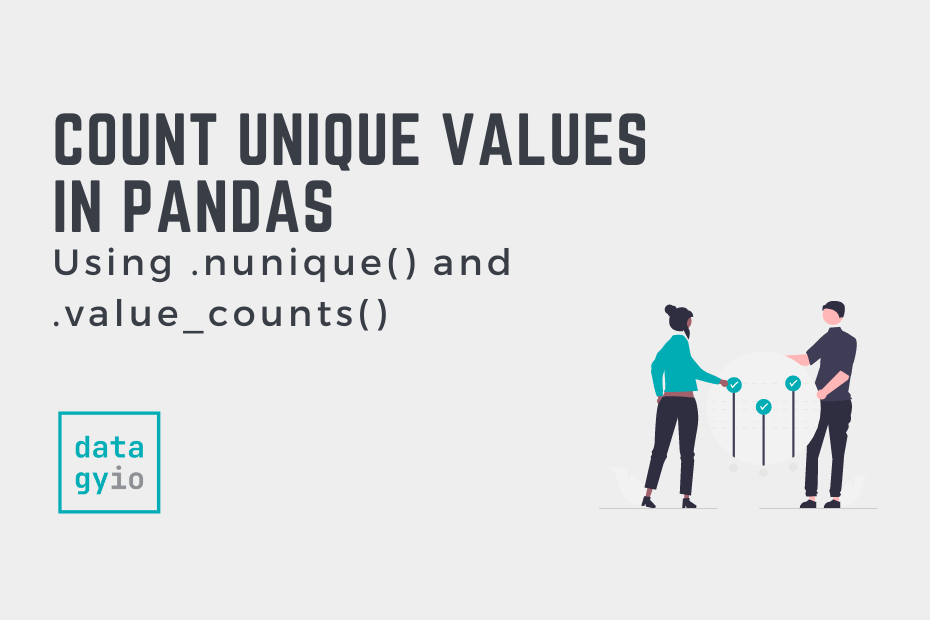# Data Analysis in Pandas## Count Unique Values in Pandas

In this tutorial, you’ll learn how to use Pandas to count unique values. You’ll learn how to count unique values in a column, in multiple columns, and in an entire DataFrame. Being able to count unique values can have important… Read More »Count Unique Values in Pandas## Summarizing and Analyzing a Pandas DataFrame

In this tutorial, you’ll learn how to quickly summarize and analyze a Pandas DataFrame. By the end of this tutorial, you’ll have learned to take on some exploratory analysis of your dataset using pandas. You’ll learn how to calculate general… Read More »Summarizing and Analyzing a Pandas DataFrame## Calculate a Weighted Average in Pandas and Python

Learn how to use Pandas to calculate the weighted average in Python, using groupby, numpy, and the zip function between two lists.## Pandas Sum: Add Dataframe Columns and Rows

Learn how to use Pandas to calculate a sum, including adding Pandas Dataframe columns and rows, and how to add columns conditionally.## Pandas Diff: Calculate the Difference Between Pandas Rows

Learn how to use the Pandas diff method to calculate the difference between dataframe rows and columns, including at defined intervals.## Normalize a Pandas Column or Dataframe (w/ Pandas or sklearn)

Learn how to normalize and standardize a Pandas Dataframe with sklearn, including max absolute scaling, min-max scaling and z-scoare scaling.## Pandas Quantile: Calculate Percentiles of a Dataframe

Learn how to use the Pandas quantile method to calculate percentiles in Pandas including how to modify the interpolation of values.## Pandas Rank Function: Rank Dataframe Data (SQL row_number Equivalent)

Learn how to use the Pandas rank method to rank you data, including how to rank a grouped dataframe using the groupby method.## Pandas Describe: Descriptive Statistics on Your Dataframe

Learn how to use the Pandas describe method to generate summary statistics on your Pandas Dataframe, including changing percentiles.## Pandas Variance: Calculating Variance of a Pandas Dataframe Column

Learn how to calculate the variance of a variable in Pandas, including how to calculate for a single column, multiple or a whole dataframe.## Pandas Mean: Calculate Pandas Average for One or Multiple Columns

Learn how to calculate the Pandas mean (or Pandas Average), including how to calculate it on a column, dataframe, and row, and with nulls.## Relative Frequencies and Absolute Frequencies in Python and Pandas

In this post, you’ll learn how to calculate relative frequencies and absolute frequencies using pure Python, as well as the popular data science library, Pandas. A relative frequency, measures how often a certain value occurs in a dataset, relative to… Read More »Relative Frequencies and Absolute Frequencies in Python and Pandas